# Quantitative Aptitude : Pipe & Cistern Tricks & Tips

## Pipe & Cistern Tricks & Tips

Problems containing pipes and cisterns fall broadly into one of two categories. The first category being one wherein questions are concerned with the time required to fill up a tank, with a combination of one or more pipes and cisterns. The second category, concerns itself with leakages and holes, requiring you to calculate the effective rate of the tank being filled or emptied.

## Type 1: Pipes and Cistern (Tank)

In order to solve questions falling into the first category, imagine each of the pipes as a human doing some work. (Again, we are using a Work and Time reference here). The time taken by a single pipe to fill up the tank by itself, is the time taken by that human to do the entire work. Find out each pipe’s (or human’s) rate of doing work, with respect to each other. For instance, if Pipe A can fill the tank in 8 hours, and Pipe B can fill it in 4 hours, you know that the rate of B is twice that of A.

Once, you have the rates of doing work, all you have to do is add them up to find out the overall rate of filling the tank by all the pipes together. Remember, rate is always inversely proportional to the time taken, so the rates you add up should be reciprocals of the time taken by each pipe.
An easy tip that helps, is to assign any one pipe’s rate as ‘x’, and calculate the others’ rate in terms of ‘x’.

E.g. 1. Pipe A fills a tank in 3½ hours whereas Pipe B fills the same tank in 10½ hours.

### Solution:

Since Pipe B takes more time, let us take ‘x’ as the rate at which B fills the tank.
Since time taken by B is 3 times the time taken by A, the rate of A will be 3 times faster than the rate of B. So the rate of A is ‘3x’.

 REMEMBER: RATE, NOT TIME!!

Another advantage of using the rates, is that rates can be added up, not time. Which means that if two pipes take 4 and 8 hours respectively to fill up a tank, you can add the reciprocals of 4 and 8 to get the net rate. What you cannot do, is add 4 and 8 up, directly. That would result in a wrong answer.
The logic is simple. If you add 4 and 8 up, to find the time taken by both together to fill up the tank, you will get 12. But think, if one pipe takes 4 hours and the other takes 8 hours to fill up the tank, they couldn’t possibly take 12 hours together! When in doubt, use this trick!

So let us use the rates in the following example:

E.g. 2. Pipes P, Q, R and S can fill a certain tank in 3, 5, 6 and 10 hours, respectively. How much time will it take for the tank to be filled if all pipes are opened together?

### Solution :

Let ‘x’ be the rate of Pipe S, which is the slowest. So the rates of all the pipes are as follows. Make sure to make as few calculations as possible – this is the trick to cracking your exam! Try the following method:

 Pipe Time Taken (in hours) Efficiency (Inverse of time) S 10 x (where x=1/10) Q 5 (1/2 of 10) 2x P 3 (1/3.33 of 10) 3.33 x R 6 (2 times 3) 1.66x (1/2 of 3.33x)

So the total efficiency of the four pipes is: x + 2x + 3.33x + 1.66x ≈ 8x = 8 × (1/10)

Thus the total time taken for the four pipes working together to fill the tank would be:

10/8 hours = 1.25 hours = 1 hour 15 mins.

By choosing the pipe taking the maximum time first and basing your calculations on that, you effectively bring down the number of reciprocal calculations you make. Of course, you need to pick and choose which pipe’s efficiency to calculate next. Like in the example, after S, we chose Q because its time taken was a direct multiple of the time taken by S. This lets us calculate its reciprocal easily. Next, we were left with Pipes P and R, both of whose times are not multiples of the time taken by S. However, we all know that 10 is 3.33 times 3. So we use this to first find the efficiency of P. Next we know that 6 is two times 3. So clearly the efficiency of R is half of P.
In Pipe And Cistern Type-1, I am going to discuss some important Pipe And Cistern Problem Shortcut Tricks .

(Q1)Two pipes A and B can fill a tank in 20 minutes and 30 minutes respectively.If both pipes are opened together, the time taken to fill the tank is.
Solution: Fig.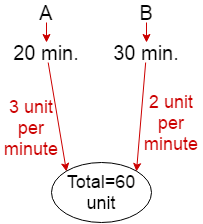Rate of Filling the tank by A and B in 1 minute=3+2=5 unit
So, total time taken by Pipe (A+B)=60/5
=12 minutes Ans.
[Concept: First step-L.C.M=20,30=60 i.e total unit. now 60/20=3 unit per minutes tank filling by pipe A and 60/30=2 unit per minutes tank filling by pipe B.]

(Q2) A tap can empty a tank in 30 minutes. A second tap can empty it in 45 minutes. If both the taps operate simultaneously, how much time is needed to empty the tank?
Solution: Fig.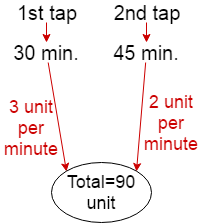Rate of emptying the tank by 1st and 2nd tap in 1 minute=(3+2)
=5 unit
So, total time taken by (1st+2nd)tap=90/5
=18 minutes Ans.

[Concept: First step-L.C.M=30,45=90 i.e total unit. now 90/30=3 unit per minutes tank emptying by 1st tap and 90/45=2 unit per minutes tank emptying by 2nd tap.]

(Q3)Three tapes A, B, C can fill an overhead tank in 4, 6 and 12 hours respectively. How long would the three taps take to fill the tank if all of them are opened together?
Solution: Fig.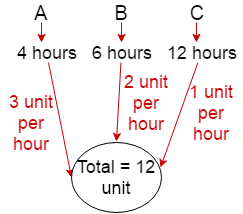Rate of Filling the tank by A, B and C in 1 hour=(3+2+1)
=6 unit
So, total time taken by (A+B+C)=12/6
=2 hours Ans.

Common Type Of Pipe And Cistern Problem Shortcut Tricks That Are Frequently Asked In Various Competitive Exam like SSC CGL, SSC CHSL, FCI etc. Type-1.1

(Q1)A water tank can filled by a tap in 30 minutes and another tap can fill it in 60 minutes. If both the taps are kept open for 5 minutes and then the first tap is closed, how much time 2nd tap will take to fill the remaining tank.
Solution: Fig.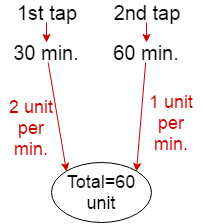Rate of Filling the tank by 1st tap and 2nd tap in 1 minute=(2+1)
=3 unit
[Concept: From fig.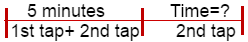According to question 1st tap and second tap को  5 minutes तक एक साथ खुला रखा गया है तो दोनों 5 minutes में कितना water fill करेगा वो निकाल लेंगे| 5 minutes के बाद तो 1st tap बंद कर दिया जा रहा है तो सिर्फ अब tank के remaining unit  को fill करने के लिए 2nd tap ही बचा है तो वो कितना time लेगा वो निकाल लेंगे. ]

Rate of Filling the tank by 1st tap and 2nd tap in 5 minute = 5×3 = 15 unit
Remaining = 60-15 = 45 unit that is filled by 2nd tap
So, total time taken by 2nd tap = 45/1 = 45 minutes Ans.

(Q2) Three pipes A, B and C can fill a tank in 6 hours, 9 hours and 12 hours respectively. B and C are opened for half an hour, then A is also opened. The time taken by the three pipes together to fill the remaining part of the tank is.

Solution: Fig.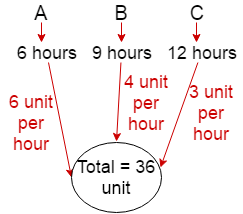Rate of Filling the tank by A+B+C in 1 hour = (6+4+3)unit  =13 unit

Concept: From fig.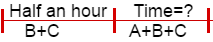half an hour तक B and C tank fill करेगा |half an hour के बाद A को open किया जाता है तो अब A, B and C मिलकर करेगा| ]
Rate of Filling the tank by B+C in 1 hour=(4+3)unit
=7 unit
Rate of Filling the tank by B+C in half an hour=7/2 unit
Remaining unit=36-(7/2)=65/2 unit
So, total time taken by (A+B+C)=(65/2)/13
=2 ½ hours Ans.
(Q3)Three pipes A, B and C can fill a tank in 6 hours. After working together for 2 hours, C is closed and A and B fill the tank in 8 hours. The time(in hours) in which the tank can be filled by pipe C alone is.
Solution:
Let tank fill by A+B+C in 1 hour= 1 unit
Tank fill by A+B+C in 6 hour= 6 unit
After working together for 2 hours then
Tank fill by A+B+C in 2 hour= 2 unit
Remaining = 6 – 2=4 unit that is filled by A and B because C is closed
Rate of filling of A+B in 1 hour=4/8=0.5 unit
Rate of filling of C in 1 hour=1-0.5=0.5 unit
Time taken by C = 6/0.5= 12 hours Ans.
Common Type Of Pipe And Cistern Problem Shortcut Tricks That Are Frequently Asked In Various Competitive Exam like SSC CGL, SSC CHSL, FCI etc. Type-1.2

(Q1)A tank has two pipes. The first pipe can fill it in 4 hours and the second can empty it in 16 hours. If two pipes are opened together at a time, then the tank will be filled in.
Solution: Fig.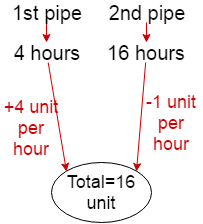Rate of filling the tank by 1st pipe= 4 unit per hour
Rate of emptying the tank by 2nd pipe= 1 unit per hour
Net rate of filling the tank=4-1=3unit
so required time=16/3=5 ¹/3 hours Ans.

[concept:जब एक pipe tank को fill(भर)कर रहा हो or दूसरा empty(खाली) कर रहा हो तो जो pipe tank को fill कर रहा होगा, तो उसका Rate of filling the tank (+ve) में लेना है or जो pipe tank को empty कर रहा होगा तो उसका Rate of emptying the tank   ( -ve) में लेना है| इसलिए figure में +ve or -ve लिया गया है.]

(Q2)Two pipes A and B can fill a cistern in 3 hours and 5 hours respectively. Pipe C can empty in 2 hours. If all the three pipes are open, in how many hours the cistern will be full?
Solution: Fig.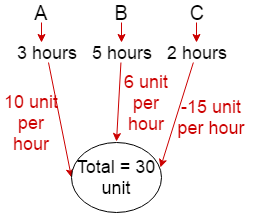Rate of filling a cistern by A= 10 unit per hour
Rate of filling a cistern by B= 6 unit per hour
Rate of emptying a cistern by C= -15 unit per hour
Net rate of filling the tank by (A+B-C)=10+6-15
=1 unit per hour
so total time taken by (A+B-C)=30/1
=30 hours Ans.

Common Type Of Pipe And Cistern Problem Shortcut Tricks That Are Frequently Asked In Various Competitive Exam like SSC CGL, SSC CHSL, FCI etc. Type-1.3

(Q1) Two pipes A and B can fill a tank with water in 30 minutes and 45 minutes respectively. Another pipe C can empty the tank in 36 minutes. First A and B are opened. After 12 minutes, C is also opened. The tank is filled up in.

Solution: Fig.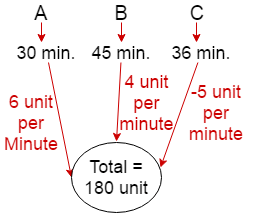Concept: From fig.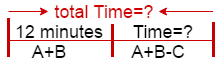Rate of filling the tank by (A+B) in 1 minute=(6+4)=10 unit
Rate of filling the tank by (A+B) in 12 minute=12×10=120 unit
Remaining=180-120=60 unit that is filled by A, B and C
Rate of emptying the tank by C = -5 unit per minute
Net rate of filling the tank by (A+B-C)=(6+4-5)
=5unit per minute
so required time taken by (A+B-C)=60/5
=12 minutes
Total time=12+12=24 minutes Ans.

Pipes and Cisterns is an important topic for banking exams. Today I am going to share technique to solve questions from this chapter quickly.

## Steps to Solve Pipes and Cistern with Trick

Step 1. Take the LCM of a given Number and that LCM will be the total capacity of cistern or
tank.Place the plus or minus sign for common understanding through picture. plus sign means time taken to fill the cistern and minus sign means to empty the cistern in a particular time.

Step 2. Add or Subtract According  to a particular question

Step 3. The LCM from Step 1. will be the total work . Divide the total capacity of cistern or tank with the outcome of Step 2.

## Examples

### #1. Two pipes A and B can fill a tank in 20 and 30 minutes respectively. If both the pipes are used together, then how long will it take to fill the tank ?(M.A.T 2003)

Step 1. Take the LCM of 20 and 30 which is 60. Sixty liter is the total capacity of tank or cistern.

Step 2. Divide 60 by (each number) 20 and 30 ,you will get 3 and 2 respectively.

Step 3. Now add  (3+2) which is 5.
Step 4. Now Divide  Total capacity of tank or cistern ( 60/5) , you will get Total time taken by them to fill the tank or cistern in a particular time which is in this case is 12 minutes.### #2.  A cistern can be filled by a tap in 4 hours while it can be emptied by another tap in 9 hours. If both the taps are opened simultaneously then after how much time will the cistern get filled ? (Hotel management, 1997)

Step 1. Take the LCM of 4 and 9 which is 36. Thirty six liter is the total capacity of tank or cistern.
Step 2. Divide 36 by (each number) 4 and 9 ,you will get 9 and 4 respectively.Here plus (+) sign means filling the tank or cistern and minus (-) implies time taken by them to empty the tank.
Step 3. Now You see one is filling the tank and other empty it so subtract it with each other.you will get (9-4) 5.
Step 4. Now Divide  Total capacity of tank or cistern ( 30/5) , you will get Total time taken by them to fill the tank or cistern in a particular time which is in this case is 36/5 minutes.### #3.

Pipe A can fill a tank in 5 hours, pipe B in 10 hours and pipe C in 30 hours. If all the pipes are open, in how many hours will the tank be filled ? (C.B.I, 1997)

Step 1. Take the LCM of 5,10 and 30 which is 30. Thirty liter is the total capacity of tank or cistern.
Step 2. Divide 30 by (each number) 5,10 and 30 ,you will get 6,3 and 1 respectively.Here plus (+) sign means filling the tank or cistern and minus (-) implies time taken by them to empty the tank.
Step 3. Now you see here each tank is filling the tank in a particular time, so add each value (6+3+1) you will get 10.
Step 4. Now Divide  Total capacity of tank or cistern ( 30/10) , you will get Total time taken by them to fill the tank or cistern in a particular time which is in this case is 3 hours.### #4.

Pipes A and b can fill a tank in 5 and 6 hours respectively. Pipe C can empty it in 12 hours. If all the three pipes are opened together, then the tank will be filled in ?(Bank P.O. 2002)## Practice Questions

### Ques 1.

Two pipes P and Q can fell a cistern in 24 min. and 36 min. respectively . Third pipe R can empty it in 36 min. If all the three pipes are opened , find the taken to fill the cistern.
(a) 1 hour
(b) 24 mins
(c) 36 mins
(d) 30 mins

### Ques 2.

A tank has a leak which would empty it in 8 hours. A tap is turned on which admit 6 liters a minute into the tank and it now emptied in 12 hours . How many literes does the tank holds?
(a) 8260 ltr
(b) 8640 ltr
(c) 8560 ltr
(d) 8800 ltr

### Ques 3.

When the waste pipe is closed , two taps can separately fill a cistern in 10 and 12 minutes respectively .when the waste pipe is opened they together fill it in 15 minutes . How long does it take waste pipe to empty the cistern , when the taps are closed?
(a) 8 min. 34 sec.
(b) 7 min.10 sec.
(c) 12 min.
(d) 10 min

### Ques 4.

Two pipes can fill a tank in 10 minutes and 30 minutes respectively and a third pipe can empty the full tank in 20 minutes . If all the three pipes are opened simultaneously, the tank will be filled in:
(a) 12 minutes
(b) 10 minutes
(c) 8 minutes
(d) 6 minutes

### Ques 5.

Two pipes can fill a cistern in 14 hours and 16 hours respectively . The pipes are opened simultaneously and it is found that due to leakage in the bottom , 32 minutes extra are taken for the cistern to be filled up. When the cistern is full , in what time will the leak empty it ?
(a) 108 hours
(b) 112 hours
(c) 116 hours
(d) 120 hours

### Ques 6.

A cistern can be filled by pipes A and B in 12 minutes and 10 minutes respectively . The full tank can be emptied by a third pipe C in 8 minutes only .If all the pipes be turned on at the same time,the cistern will be full in :
(a) 17 min
(b) 171/7 min.
(c) 17 2/7 min.
(d) 18 min.

### Ques 7.

If two pipes function simultaneously , the reservoir will be filled in 12 hours. One pipe fills the reservoir 10 hours faster than the other. How many hours does it take the second pipe to fill the reservoir?
(a) 20 hours
(b) 25 hours
(c) 30 hours
(d) 40 hours

### Ques 8.

A tank is filled in hours by three pipes A, B and C . The pipe C is twice as fast as B and B is twice as fast as A. How much time will pipe A alone take to fill the tank?
(a) 40 hours.
(b) 35 hours
(c) 30 hours
(d) 25 hours

### Ques 9.

Three pipes P , Q and R can fill a tank in 6 hours , After working it at together for 2 hours , R is closed and P and Q can fill the remaining part in 7 hours . How much time will R take alone to fill the tank?
(a) 16 hours
(b) 14 hours
(c) 12 hours
(d) 10 hours

### Ques 10.

Three taps P, Q and R can fill a tank in 12, 15 and 20 hours respectively . If P is opened all the time and Q and R are opened for one hour each alternately , the tank will be full in
(a) 5 hours
(b) 6 hours
(c) 6 2/3 hours
(d) 7 hours

### Solutions:

Ans 1.Since time taken to fill the cistern by Q = time taken to empty the cistern by R so
the cistern will be filed by P in 24 min.

Ans 2. In I hour the part filled by the tap = 1/8 -1/12 = 1/24
Hence , the tap can fill the tank in 24 hours.
Therefore, capacity of the tank = 24 x 60 x 6 = 8640 litres.

Ans 3. The waste pipe can empty in 1 min .= 1/10 + 1/12 – 1/15 = 11/60 – 1/15 = 7/60
of the cistern.
Hence, the waste pipe can empty the cistern in 60/7 minutes , i.e., 8 minutes 34 sec.

Ans 4. 1n I minute the part filled by all the three pipes = 1/10 + 1/30 – 1/20 = 1/12
Hence , all the three pipes will fill the bank in 12 minutes.

Ans 5. In I hour the part filled by both the pipes = 1/14 + 1/16 = 15/112
Hence, both the pipes will fill the cistern in 112/15 min.
but due to leakage , the tank will be filled in 112/15 + 32/60 = 120/15 = 8 hours.
Since, in 1 hr. the part emptied by the leakage = 15/112 – 1/8 = 1/112
Therefore , leakage will empty the tank in 112 hours.

Ans 6. In 1 minute the part filled by the three pipes = 1/12 + 1/10 -1/8 = 7/120
Hence, all the three pipes will fill the cistern in 120/7 = 17 1/7 minutes.

Ans 7.Let ( x- 10) and x hrs be the time taken by two pipes to fill the reservoir , then,Hence , second pipe will fill the reservoir in 30 hours.

Ans 8.Let x,x/2 and x/4 hours be the time taken by pipes A, B and C respectively to
fill the tank,then
1/x + 2/x + 4/x = 1/5 => 7/x = 1/5            ∴ x = 35 hours.
Hence , time taken by A is 35 hours to fill the tank.Ans 9. In 2 hour the part filled by all the three pipes = 2 X 1/6 = 1/3
Remaining part = 1 – 1/3 = 2/3, which is filled by ( P + Q ) in 7 hours.
Hence , in 1 hour the part filled by ( P + Q ) = 2/ 3 x 7 = 2/21
Since , in 1 hour the part filled by R alone = 1/6 – 2/21= 1/14
Therefore, R alone will fill the bank in 14 hours.Ans 10.In 1 hour the part filled by (P + Q ) = 1/12 + 1/15 = 3/20
In 1 hour the part filled by (P + R ) = 1/12 + 1/20 = 2/15
Hence, in 2 hours the part filled by ( P+ Q +R ) = 3/20 + 2/15 = 17/60
Then , in 6 hours the part filled by ( P + Q + R ) = 3 x 17/60 = 17/20
Remaining part = 1 – 17/20 = 3/20 , which is filled by ( P + Q ) in 1 hour.
Hence, total time taken = 6 + 1 = 7 hours.

## CISTERN:

Cistern is referred to a tank/Reservoir which has some specific capacity to hold liquid.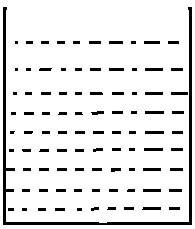## PIPES: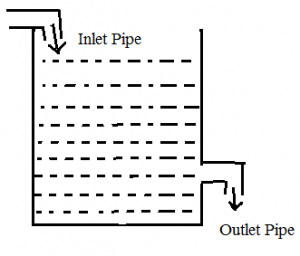There are two types of Pipes,They are
1)Inlet Pipe
2)Outlet Pipe
Inlet Pipe:
Inlet Pipe is used to fill the tank.
Outlet Pipe:
Outlet Pipe is used to empty the tank.
There may be any number of Inlet Pipes and Outlet Pipes that are connected to a cistern.
Before Getting into this topic,let us remember 3 basic terms
Total Capacity=LCM(Given Numbers)(unit-Litres)
Time=Total Capacity/Efficiency(unit-hr,sec,min,day)
Efficiency=Total Capacity/Time(unit Litre/hour)

## #.1.TYPE 1: PROBLEMS BASED ON INDIVIDUAL CAPACITY:

There are 3 sub type in this type,they are
1. When both the pipes are Inlet Pipes
2. When both the pipes are Outlet Pipes
3. One Pipe is Inlet Pipe and Outlet Pipe

### 1) When both the pipes are Inlet Pipes:

1)Pipe A can fill the tank in 10 hrs and Pipe B can fill the tank in 40 hrs.How long will it take to fill the tank if both the pipes are opened?
Explanation
Given that,
Pipe A can fill the tank in 10 hrs and Pipe B can fill the tank in 40 hrs
Total Capacity=LCM(10,40)=40Litres
A’s Efficiency=40/10=4 litre/hour
B’s Efficiency=40/40=1 litre/hour
So,
When both are opened,their total capacity=4+1=5 Litre/hour
Time=40/5=8 hours
So,When Pipe A and B are opened together,they can fill the tank in 8 hours

### 2) When both the pipes are Outlet Pipes:

1)Pipe A can empty the tank in 3 hours where as Pipe B can empty the tank in 6 hours.When they opened together how,long will it take to empty the tank?
Explanation
Given that,
Pipe A can empty the tank in 3 hours where as Pipe B can empty the tank in 6 hours.
Total Capacity of the tank=LCM(3,6)=6 Litres
A’s Efficiency=6/3=2litres/hour
B’s Efficiency=6/6=1 litre/hour
Total Efficiency of (A+B)=(2+1=3litres/hour)
Time=6/3=2hours
Therefore,When A & B are opened together,they can empty the tank in 2 hours

### 3) One Pipe is Inlet Pipe and Outlet Pipe:

1)A can fill the tank in 12 hours,B can empty the tank in 15 hours.When both the pipes are opened find how long will it take to empty/fill the tank ?
Explanation
Given that,
A can fill the tank in 12 hours,B can empty the tank in 15 hours
Total Capacity=LCM(12,15)=60 Litres
A’s(Inlet Pipe) Efficiency=60/12=5litre/hour
B’s(Outlet Pipe)Efficiency=60/15=4litre/hour
Note:
If the Efficiency of Inlet Pipe is greater then the Outlet Pipe the tank will be filled,
If the Efficiency of Outlet Pipe is greater then the Inlet Pipe then the tank will be Emptied
Inlet Pipe fill 5 litre in 1 hour at the same time Outlet Pipe empty 4 Litre in 1 hour so,in 1 hour only 1 litre is filled in the tank.
Time taken to fill the tank=60/1=60hours

## #.2.TYPE 2: BASED ON PARTIAL WORK:

1)Pipe A can fill the tank in 15 minutes and Pipe B can fill the tank in 20 Minutes.They opened together for 4 Minutes and after that B was turned off.How long will A take to fill the Remaining tank capacity?
Explanation
Given that,
Pipe A=15 minutes(fill)
Pipe B=20 minutes(fill)
Total Capacity=LCM(15,20)=60litres
Efficiency of A=60/15=4litre/min
Efficiency of B=60/20=3litre/min
They were Opened for 4 Minutes,
4+3=7litre/min
7*4=28 Litre.
Remaining 60-28=32 litre
After that B was turned off,then 32 litre is filled by Pipe A
=32/4=8 min

## #.3. TYPE 3: BASED ON EFFICIENCY

1)A is thrice as efficient as B,If both the pipes are opened together the tank is filled in 2 days,then how long will it take to fill the tank only if Pipe B is Opened?
Explanation
Let us Assume the capacity of Pipe B=10litre/day
Therefore,Capacity of Pipe A=3(B)
=3(10)=30litre/day
So When they are opened together,they can fill 40 litres/day
In 2 days,they can fill 80litres
If Pipe B is Opened then it will take-80/10=8 days

## #.4. TYPE 4 : BASED ON TIME

1)There are 4 inlet Pipes namely A,B,C,D and their individual capacity to fill the tank is 20 hours,30 hours,40 hours and 50 hours respectively.A opens at 6.00 A.M.,B opens at 8.00 A.M.,C opens at 9 A.M. and D opens at 10 A.M.Find at what time the tank will be filled?
Explanation
Total Capacity=LCM(20,30,40,50)=600 Litres
A’s Efficiency=600/20=30litres/hour
B’s Efficiency=600/30=20litres/hour
C’s Efficiency=600/40=15 litres/hour
D’s Efficiency =600/50=12 litres/hour
Given that,
A is opened at 6.00 A.M. till 10.00 A.M,it is opened for 4 hours
=30*4=120 litres/hour
B is opened at 8.00 A.M. till 10 A.M.,it is opened for 2 hours
=20*2=40 litres/hour
C is opened at 9.00 A.M. till 10 A.M., it is opened for 1 hour
=15*1=15 litres/hour
At 10.00 A.M
Three Pipes (A,B & C fill 175 litres)
Remaining Capacity in the tank=600-175=425 litres
This 475 litres is filled by all the 4 Inlet Pipes
Time=475/(30+20+15+12)
=475/77
=5 hours 30 Minutes
So from 10 A.M. it would take 5 hours 30 Minutes
The tank will be filled at 3.30 P.M

## #.5 TYPE 5:

### BASED ON PARTIAL TIME:

1)Two Pipes A & B fill the tank in 60 Minutes and 40 Minutes respectivley.How many minutes will it take to fill the tank from empty state if B is used for half the time & A and B used to fill Other half?
Explanation
Let the Total Capacity=LCM(60,40)=120 Litres
Efficiency of A=120/60=2 litre/minute
Efficiency of B=120/40=3 litre/minute
Let the time taken by the pipes to fill the tank be X
Half the time=X/2 is used to fill the tank by B & Another half the time is used to fill the tank by A+B
=X/2(Filled by Pipe B)+X/2(Filled by Pipe A+B)
=(X/2)* (3) + (X/2)* (5)
=3(X/2) + 5(X /2)
=8(X/2)
We know that
Total Capacity=Time * Efficiency
120 = (X/2) * 8
240=X*8
30=X
Therefore, the tank is filled in 30 Minutes

What a typical question looks like?
In such questions, basically a tank has to be filled by two (or more) pipes and we are given:

1) Time taken by each pipe to fill the tank.

2) Total time taken to fill the tank

We’re, usually, given either of the aforementioned information & we’ve to find out the other.
The problem with maximum difficulty asked for Pipes & Cistern can be described in the image below:Here, we’ve a ‘Tank’ around which the whole question revolves. Basically, we’ve to find out in how long the whole tank could be filled or emptied. Then there are Inlet Pipes (A and B),there can any number of Inlet pipes.
Inlet pipes are responsible for filling the tank. They, basically, bring the water inThe workdone by them is positive.
Then we an Outlet pipe, there can be any number of outlet pipes too. Outlet pipes are responsible for emptying the tank. They, basically, put the water out. The work done by them is negative.

Rules for solving such questions:

1. If a pipe can fill the tank in ‘x ’ hours then, the part filled in 1 hour = 1/x

2. If a pipe can empty the tank in ‘ y’ hours then, the part emptied in 1 hour = 1/y

3. If a pipe can fill the tank in ‘x ’ hours  and another can empty it in ‘y’ hours then, the net part filled in 1 hour = 1/x – 1/y ; Total time taken to fill such tank = xy/y-x

4. If three such pipes are there where ‘x’, ‘y’ are inlet and ‘z’ is outlet, Total Time =5. A pipe can fill the tank in ‘x’ hrs. Due to leak it is filled in ‘y’ hrs, time taken by leak to empty the tank =xy/y – x hrs
6. If leak time > Inlet pipe then tank will be filled; If leak time < Inlet pipe then tank will be emptied.

Sample Questions:
Qs. 1 – Pipe A can fill the tank in 20 hours while Pipe B alone can fill it in 30 hours and Pipe C can empty the tank in 40 hours. If all the pipes are opened together, in how long will the tank be full?

Solutions – Net part filled in 1 hour  = 1/20 + 1/30  – 1/40 (as work done by C is negative)
= 7/120
⇒ Full tank will be full in  120/7 = 17  1/7 hours.

Q2. There’s a leak in the bottom of tank. When the tank is thoroughly repaired, it would be filled in 3.5 hours. It now takes half an hour longer. If tank is full, how long would it take to leak the tank?

Sol. Here, clearly the ‘leak’ is working like an Outlet pipe.

Done using rule 5)
We need to find the time taken to empty tank by leak (or outlet pipe) if tank is full
Repaired tank is filled in 3.5 hours ⇒ Inlet pipe takes 3.5 hours
Un-repaired tank takes 3.5+0.5 = 4 hrs ⇒ time  taken 4 hours to fill tank.
Total time taken to empty such tank = xy/y-x = 3.5 x 4 / 4 – 3.5 = 28 hrs.
Leak would empty the cistern in 28 hours.

Q3.Two pipes P and Q would fill tank in 24 hours and 32 hrs respectively. If both pipes are opened together, find when the first pipe must be turned off so that the tank may be just filled in 16 hrs?

Sol. Suppose the pipe P is closed after ‘x’ hours.
Then, P pipe would fill in 1 hr = 1/24 and in x hrs = x / 24

Pipe Q would fill in 1 hour =  1 / 32 and in 16 hrs (as tank is full in 16 hrs) = 16 /32 = 1/2

Pipe P work in ‘x’ hr + Pipe Q work in 16 hrs = 1 (as they complete the 1 unit of work) = x/24 + 16/32 = 1
⇒ x = 12 hours.

Short method:
The first pipe should work for =Q4. Three pipes A, B and C can fill cistern in 6 hrs. After working together for 2 hrs, C is closed and A & B fill it in 8 hrs. Then find the time in which cistern can be filled by pipe C.

Sol: A + B + C  work in 1 hr = 1/6 of cistern
A+B+C work in 2 hr = 1/6
A+B+C work in 2 hr = 1/6 × 2 = 1/3 of cistern
Unfilled part after 2 hrs = 1 – 1/3 = 2/3 of Cistern
This 2/3 of cistern is filled by A & B in 8 hrs.
⇒ A & B can fill the full cistern in = 8×3/2 = 12 hrs
We know that A+B+C = 6 hrs
C = (A+B+C) – (A+B) = (1/6) – (1/12) = 1/12

⇒ C alone would fill it in 12 hrs.

Q5. A tank has a leak which would empty it in 8 hrs. A tap is turned on which admits 6 liters a minute into tank, and it’s now emptied in 12 hrs. How many liters does the tank hold?

Sol. Time by Outlet Pipe = 8 hrs
Tank emptied in = 12 hrs

Done using rule 5)

Time by Inlet pipe = (12×8)/(12 – 8) = 24 hrs.
Also given: Inlet pipe takes 6 liters in a minute ⇒ In 1 hr, intake = 6×60 = 360L
⇒ Intake in 24 hrs = 360×24 = 8640 liters
Hence, the total capacity of tank is 8,640 L.

Note: If it’s given that tank takes 8 hrs to get full but with leak it takes 2 hrs more, then 8 hrs is the time taken by Inlet pipe and 10 hrs is total time to fill with leak.

Qs. 6A can fill tank in 12 minutes, B in 15 minutes and C empties it in 6 minutes. A and B are opened for 5 minutes then C is also opened. In what time is the tank empty?

Sol. A + B in 5 minutes = [1/12 + 1/15]×5 = ¾
⇒ 3/4th part of tank is filled in 5 minutes.
When C is also opened, work done by all pipes in 1 minute = 1/12 + 1/15 – 1/6 = 1/60

When all three are opened, the tank is emptied in 60 minutes.
So,  3/4 part will be emptied in = 60 x 3/4 = 45 minutes

Q7. Two pipes can separately fill a tank in 20 hrs and 30 hrs respectively. Both the pipes are opened to fill the tank but when tank is 1/3 full a leak is developed in the tank through which 1/3 of water supplied by both the tank leak out. What is total time taken to fill the tank?

Sol. Time taken by two pipes to fill the tank = (20×30)/(20+30) = 12 hrs.
1/3rd tank is filled in = 12×1/3 = 4 hrs; Left time = 12 – 4 = 8 hrs.

Now, leakage develops which empties 1/3rd of water supplied (by both pipes)
⇒ Now, efficiency of Inlet pipes = 1 – 1/3 = 2/3rd .

Earlier, at 1 efficiency they were taking 8 hrs
now at 2/3rd efficiency they will take 8 ÷ 2/3 = 12 hrs

⇒ Total time taken to fill the tank= 4 + 12 = 16 hrs